## Text Detector for OCR

OCR(Optical Character Recognition) consists of text localization + text recognition. (text localization finds where the characters are, and text recognition reads the letters.)

This text detector acts as text localization and uses the structure of RetinaNet and applies the techniques used in textboxes++.

After performing localization, each text area is cropped and used as input for text recognition. An example of text recognition is typically the CRNN.

Combining this text detector with a CRNN makes it possible to create an OCR engine that operates end-to-end.

This text detector is implemented in two frameworks, pytorch and tensorflow, and multi scale training is only possible in pytorch implementations.

## How to use

• single scale training
``````#step1 : SynthText, 608x608
CUDA_VISIBLE_DEVICES=0,1 python train.py --dataset=SynthText --batch_size=16 --input_size=608 --logdir=logs/single_step1/ --save_folder=weights/single_step1/ --num_workers=6

#step2 : ICDAR2013 / 2015, 768x768
CUDA_VISIBLE_DEVICES=0,1 python train.py --dataset=ICDAR2015 --batch_size=16 --input_size=768 --logdir=logs/single_step2/ --save_folder=weights/single_step2/  --num_workers=6 --resume=weights/single_step1/ckpt_40000.pth
CUDA_VISIBLE_DEVICES=0,1 python train.py --dataset=ICDAR2013/MLT --batch_size=16 --input_size=768 --logdir=logs/single_step2/ --save_folder=weights/single_step2/  --num_workers=6 --resume=weights/single_step1/ckpt_40000.pth

#step3 : ICDAR2013 / 2015, 960x960
CUDA_VISIBLE_DEVICES=0,1 python train.py --dataset=ICDAR2015 --batch_size=8 --input_size=960 --logdir=logs/single_step3/ --save_folder=weights/single_step3/  --num_workers=6 --resume=weights/single_step2/ckpt_10000.pth
``````
• multi scale training (only pytorch)
``````#step1 : SynthText, MS(608x608 ~ 960x960)
CUDA_VISIBLE_DEVICES=0,1 python train.py --dataset=SynthText --batch_size=12 --multi_scale=True --logdir=logs/multi_step1/ --save_folder=weights/multi_step1/ --num_workers=6

#step2 : ICDAR2013 / 2015, MS(608x608 ~ 960x960)
CUDA_VISIBLE_DEVICES=0,1 python train.py --dataset=ICDAR2015 --batch_size=12 --multi_scale=True --logdir=logs/multi_step2/ --save_folder=weights/multi_step2/ --num_workers=6 --resume=weights/multi_step1/ckpt_40000.pth
CUDA_VISIBLE_DEVICES=0,1 python train.py --dataset=ICDAR2013/MLT --batch_size=12 --multi_scale=True --logdir=logs/multi_step2/ --save_folder=weights/multi_step2/ --num_workers=6 --resume=weights/multi_step1/ckpt_40000.pth

#step3 : ICDAR2013 / 2015, MS(960x960 ~ 1280x1280)
CUDA_VISIBLE_DEVICES=0,1 python train.py --dataset=ICDAR2015 --batch_size=6 --multi_scale=True --logdir=logs/multi_step3/ --save_folder=weights/multi_step3/ --num_workers=6 --resume=weights/multi_step2/ckpt_10000.pth
``````
• For Evaluation
``````CUDA_VISIBLE_DEVICES=0 python eval.py  --input_size=1280 --nms_thresh=0.1 --cls_thresh=0.4 --dataset=ICDAR2015 --tune_from=weights/multi_step3/ckpt_4000.pth --output_zip=multi_step3_zip
``````

## TextBoxes++

• SSD structure is used, and vertical offset is added to make bbox proposal.
• The structure is the same as TextBoxes, but the offset for the QuadBox has been added.
• 4d-anchor box(xywh) offset -> (4+8)-d anchor box(xywh + x0y0x1y1x2y2x3y3) offset
• last conv : 3x5 -> To have a receptive field optimized for the quad box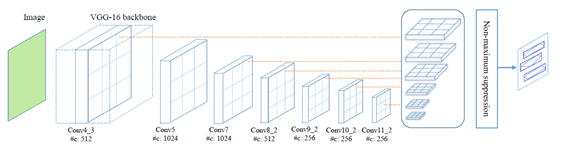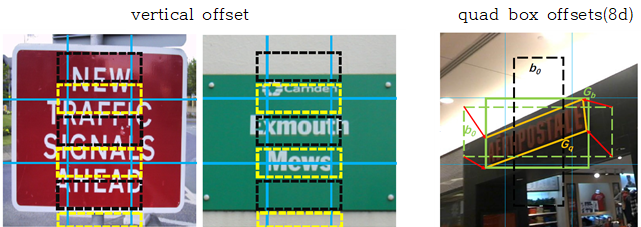## RetinaNet

• Simple one-stage object detection and good performance
• FPN (Feature Pyramid Network) allows various levels of features to be used.
• output : 1-d score + 4d-anchor box offset
• cls loss = focal loss, loc loss = smooth L1 loss
• ImageNet pre-trained weight initialize required! -> loss explode, just can not learn!
• batch norm freeze is also required! If you do not freeze, your learning will not work.
• Freeze BN or Group Norm works well. However, GN was slow (group = 32) and there was no significant performance difference with freeze BN.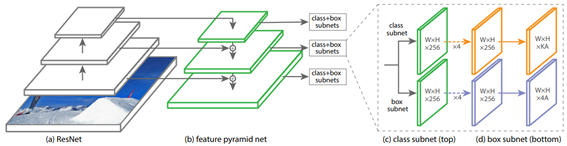## Encode

1. Define anchor boxes for each grid.
2. Obtain the IoU between the GT box and the anchor box.
3. Each anchor box is assigned to the largest GT box with IoU.
4. At this time, IoU> 0.5: Text (label = 1) / 0.4 <IoU <0.5: Ignore (label = -1) / IoU <0.4: non-text (label = 0).
5. Perform anchor box coordinate encoding using the following formula.

## Results

Framework Dataset Hmean backbone input size training scale cls thresh nms thresh iter weights
Tensorflow ICDAR2013 0.8107 se-resnet50 768x768 960x960 0.3 0.1 Synth(62k) + IC15(17k) link
Tensorflow ICDAR2015 0.7916 se-resnet50 1280x1280 960x960 0.3 0.1 Synth(62k) + IC15(17k) link
Pytorch ICDAR2013 0.8298 se-resnet50 multi Multi scale 0.5 0.35 Synth(35k) + IC13+MLT(7k) link
Pytorch ICDAR2015 0.8065 se-resnet50 1280x1280 Multi scale 0.4 0.20 Synth(35k) + IC15(4k) link

## Good case

• It responds well to the quad box and finds boxes of various sizes.

• Red : Prediction / Green : GT / Yellow : Don't Care

• ICDAR2013• ICDAR2015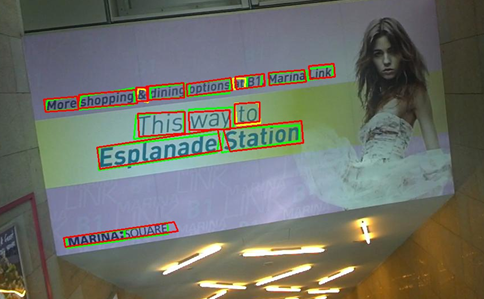## Bad case

• It is weak in vertical box and long text ..!

• Compared to the GT box, there is not enough fitting to wrap the text area well.

• It is vulnerable to ambiguous text or first-seen text.

• Red : Prediction / Green : GT / Yellow : Don't Care

• ICDAR2013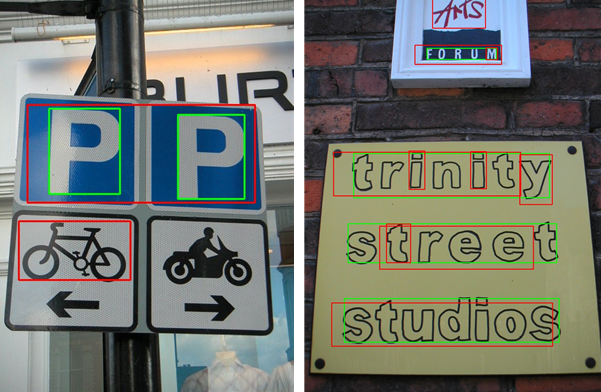• ICDAR2015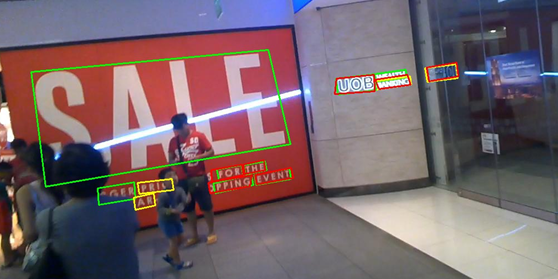## Experiments

• v1 : 2-classes, focal loss, loc=12-d, SGD, loss alpha=0.2, FPN [C3-C5] , anchor scale [32x32-512x512]
• v2 : 1-class, focal loss, loc=12-d, SGD, loss alpha=0.2, FPN [C3-C5] , anchor scale [32x32-512x512]
• v3 : 2-classes, focal loss, loc=8-d, SGD, loss alpha=0.5, FPN [C3-C5] , anchor scale [32x32-512x512]
• v4 : 1-class, focal loss, loc=8-d, SGD, loss alpha=0.5, FPN [C3-C5] , anchor scale [32x32-512x512]
• v5 : 1-class, focal loss, loc=8-d, SGD, loss alpha=0.5, FPN [C2-C5] , anchor scale [16x16-512x512]
• v6 : 1-class, focal loss, loc=8-d, SGD, loss alpha=0.5, FPN [C2-C5] , anchor scale [30x30-450x450]

## Todo list:

• [x] validation infernece image visualization using Tensorboard
• [x] add augmentation ( + random crop)
• [x] Trainable BatchNorm -> Not work!
• [x] Freeze BatchNorm -> work!
• [x] GroupNorm -> work!
• [x] add vertical offset
• [x] make SynthText tfrecord
• [x] make ICDAR13 tfrecord
• [x] make ICDAR15 tfrecord
• [x] add evaluation code (IC13, IC15)
• [x] use SE-resnet backbone
• [x] (Experiment) change anchor boxes scale half size -> worse result
• [x] (Experiment) FPN use C2~C5 -> little improvement
• [x] 1-class vs 2-classes -> no big difference
• [x] (binary) focal loss vs OHEM+softmax -> fail
• [x] QUAD version NMS (numpy version)
• [x] QUAD version Random Crop
• [x] (try) loc head : 4+8 -> 8 -> no big difference
• [x] Multi-scale training (only pytorch)
• [x] get anchor boxes using K-means clustering
• [x] change upsample function for 600 input (only pytorch)

## Environment

• os : Ubuntu 16.04.4 LTS
• GPU : Tesla P40 (24GB)
• Python : 3.6.6
• Tensorflow : 1.10.0
• Pytorch : 0.4.1
• tensorboardX : 1.2
• CUDA, CUDNN : 9.0, 7.1.3